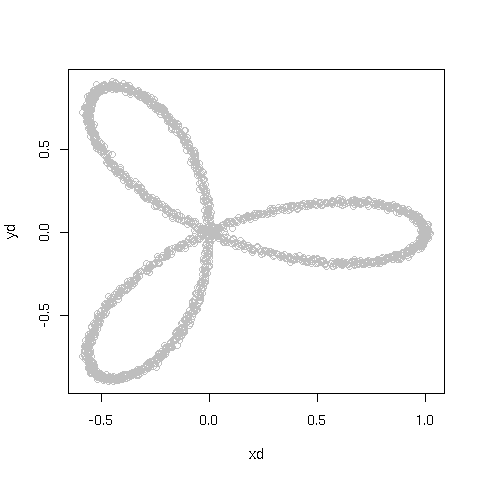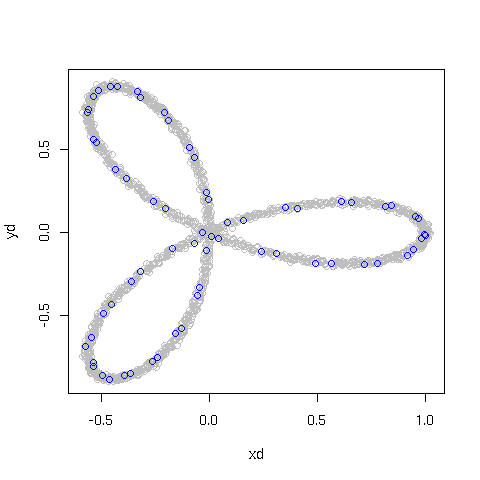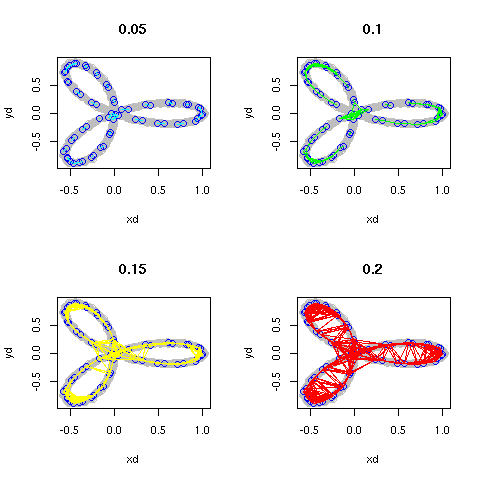# R and topological data analysis

Published: Sat 23 August 2008

This is extremely early playing around. It touches on things I'm going to be working with in Stanford, but at this point, I'm not even up on toy level.

We'll start by generating a dataset. Essentially, I'll take the trefolium, sample points on the curve, and then perturb each point ever so slightly.

```idx <- 1:2000
theta <- idx*2*pi/2000
a <- cos(3*theta)
x <- a*cos(theta)
y <- a*sin(theta)
xper <- rnorm(2000)
yper <- rnorm
xd <- x + xper/100
yd <- y + yper/100
cd <- cbind(xd,yd)
```
As a result, we get a dataset that looks like this:So, let's pick a sample from the dataset. What I'd really want to do now would be to do the witness complex construction, but I haven't figured enough out about how R ticks to do quite that. So we'll pick a sample and then build the 1-skeleton of the Rips-Vietoris complex using Euclidean distance between points. This means, we'll draw a graph on the dataset with an edge between two sample points whenever they are within ε from each other.

So we pick a sample from this sample. Every 31 points might be good. (number arrived at by guessing wildly, and drawing the resulting images until they looked pretty enough)

```csamp <- cd[seq(1,dim(csamp),31),]
```
We'd get, from this, the following result:Now, we'll want to build the corresponding skeleton. Let's do it for a few different εs to demonstrate the difference.

```d <- function(x,y,z,w) { sqrt((x-z)^2+(y-w)^2) }
par(mfrow=c(2,2))
eps <- c(0.05,0.1,0.15,0.2)
cols <- c("cyan","green","yellow","red")
for (ei in 1:length(eps)) {
plot(cd,col="gray")
points(csamp,col="blue")
title(eps[ei])
for (i in 1:dim(csamp)) {
for (j in 1:dim(csamp)) {
x <- csamp[i,1]; y <- csamp[i,2]
z <- csamp[j,1]; w <- csamp[j,2]
e <- eps[ei]
if(d(x,y,z,w) < 2*e) {
segments(x,y,z,w,col=cols[ei])
} else {
}
}
}
}
```
The result is:We notice that as the radius we observe grows, we connect all the loops, but by the time the loops are completely connected, there are also cross connections forming toward the middle. However, with any luck, these cross connections will be so short-lived, in terms of the radii we use, so that the homology classes we extract from the Rips-Vietoris complexes are noticably more persistent.

Doing the corresponding computation relies on me figuring enough out to use the Plex software suite, or writing my own, and thus will be subject of a much later blog post. This one was mainly "Look - I use R" and "Look - pretty pictures". Enjoy.